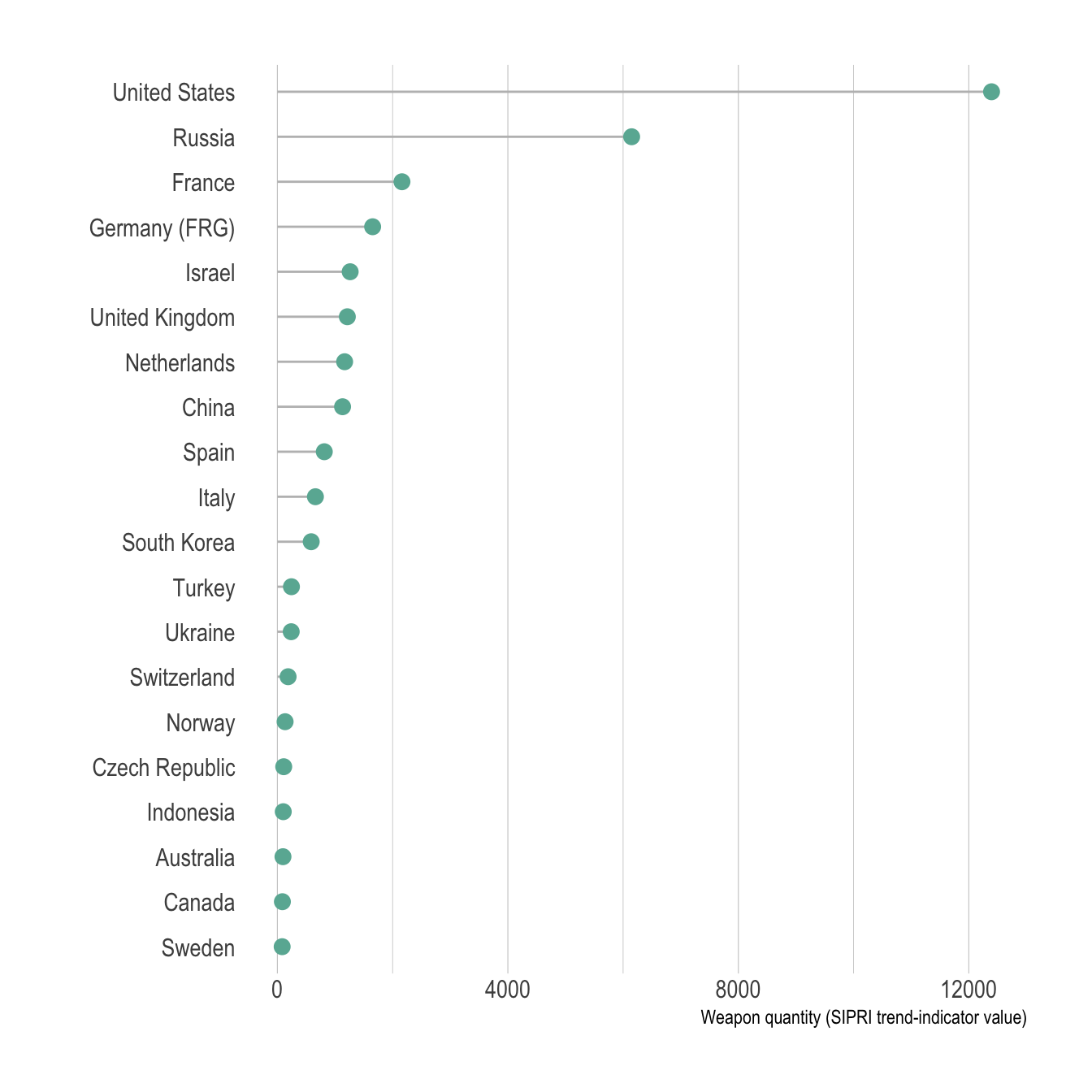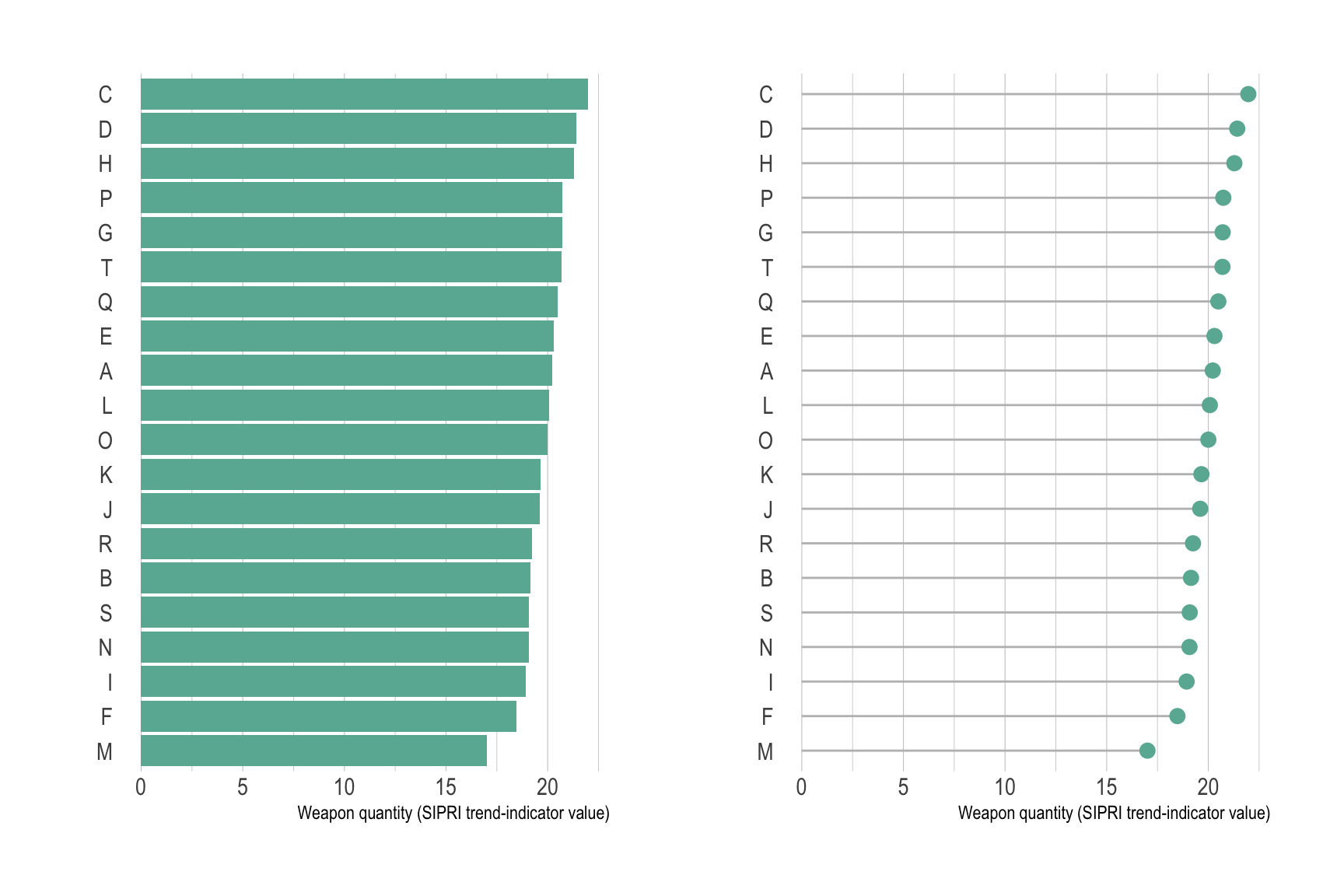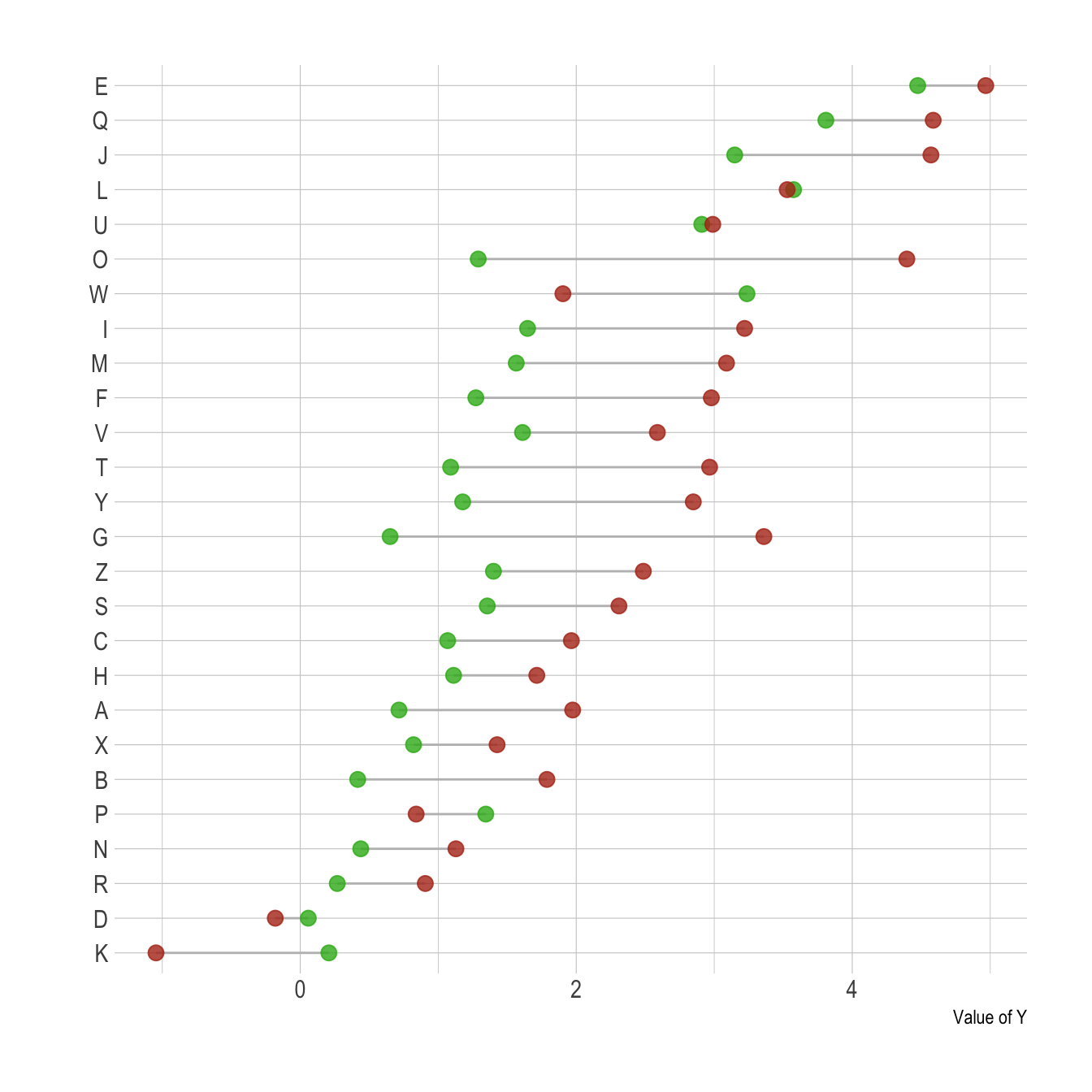# Definition

A `lollipop plot` is basically a barplot, where the bar is transformed in a `line` and a `dot`. It shows the relationship between a numeric and a categoric variable.

Here is an example showing the quantity of weapons exported by the top 20 largest exporters in 2017 (more info here):

``````# Libraries
library(tidyverse)
library(hrbrthemes)
library(kableExtra)
options(knitr.table.format = "html")
library(patchwork)

# Load dataset from github

# Plot
data %>%
filter(!is.na(Value)) %>%
arrange(Value) %>%
tail(20) %>%
mutate(Country=factor(Country, Country)) %>%
ggplot( aes(x=Country, y=Value) ) +
geom_segment( aes(x=Country ,xend=Country, y=0, yend=Value), color="grey") +
geom_point(size=3, color="#69b3a2") +
coord_flip() +
theme_ipsum() +
theme(
panel.grid.minor.y = element_blank(),
panel.grid.major.y = element_blank(),
legend.position="none"
) +
xlab("") +
ylab("Weapon quantity (SIPRI trend-indicator value)")``````# What for

The lollipop plot is used exactly in the same situation than a barplot. However it is somewhat more appealing and convey as well the information. It is especially useful when you have several bars of the same height: it avoids to have a `cluttered` figure and a Moiré effect.

``````don <- data.frame(
group = LETTERS[1:20],
val = 20 + rnorm(20)
)

p1 <- don %>%
arrange(val) %>%
mutate(group=factor(group, group)) %>%
ggplot( aes(x=group, y=val) ) +
geom_bar(stat="identity", fill="#69b3a2") +
coord_flip() +
theme_ipsum() +
theme(
panel.grid.minor.y = element_blank(),
panel.grid.major.y = element_blank(),
legend.position="none"
) +
xlab("") +
ylab("Weapon quantity (SIPRI trend-indicator value)")

p2 <- don %>%
arrange(val) %>%
mutate(group=factor(group, group)) %>%
ggplot( aes(x=group, y=val) ) +
geom_segment( aes(x=group ,xend=group, y=0, yend=val), color="grey") +
geom_point(size=3, color="#69b3a2") +
coord_flip() +
theme_ipsum() +
theme(
panel.grid.minor.y = element_blank(),
panel.grid.major.y = element_blank(),
legend.position="none"
) +
xlab("") +
ylab("Weapon quantity (SIPRI trend-indicator value)")

p1 + p2``````# Variation

The `Cleveland dotplot` is a handy variation, allowing to compare the value of 2 numeric values for each group. This kind of data could also be visualized using a grouped or stack barplot. However, this representation is less cluttered and way easier to read. Use it if you have 2 subgroups per group.

``````# Create data (could be way easier but it's late)
value1 <- abs(rnorm(26))*2
don <- data.frame(
x=LETTERS[1:26],
value1=value1,
value2=value1+1+rnorm(26, sd=1)
) %>%
rowwise() %>%
mutate( mymean = mean(c(value1,value2) )) %>%
arrange(mymean) %>%
mutate(x=factor(x, x))

# With a bit more style
ggplot(don) +
geom_segment( aes(x=x, xend=x, y=value1, yend=value2), color="grey") +
geom_point( aes(x=x, y=value1), color=rgb(0.2,0.7,0.1,0.8), size=3 ) +
geom_point( aes(x=x, y=value2), color=rgb(0.7,0.2,0.1,0.8), size=3 ) +
coord_flip()+
theme_ipsum() +
theme(
legend.position = "none",
panel.border = element_blank(),
) +
xlab("") +
ylab("Value of Y")``````Note: The term `cleveland dotplot` does not look to be very well defined as far as I know, and looks to be sometimes used for dotplots or classic lollipop plots as well. The previous chart is also called Dumbbell dot plots. Further investigation is needed on this matter and any feedback is more than welcome.

Note that with a number of subgroups between 3 and ~7 this type of lollipop plot is nice as well:

``````# Create data (could be way easier but it's late)
value1 <- abs(rnorm(6))*2
don <- data.frame(
x=LETTERS[1:24],
val=c( value1, value1+1+rnorm(6, 14,1) ,value1+1+rnorm(6, sd=1) ,value1+1+rnorm(6, 12, 1) ),
grp=rep(c("grp1", "grp2", "grp3", "grp4"), each=6)
) %>%
arrange(val) %>%
mutate(x=factor(x, x))

# With a bit more style
ggplot(don) +
geom_segment( aes(x=x, xend=x, y=0, yend=val), color="grey") +
geom_point( aes(x=x, y=val, color=grp), size=3 ) +
coord_flip()+
theme_ipsum() +
theme(
legend.position = "none",
panel.border = element_blank(),
panel.spacing = unit(0.1, "lines"),
strip.text.x = element_text(size = 8)
) +
xlab("") +
ylab("Value of Y") +
facet_wrap(~grp, ncol=1, scale="free_y")``````# Common mistakes

• Order your groups. If the levels of your categoric variable have no obvious order, order the bars following their values.

• If for whatever reason your bars must remain unsorted, it is probably better to use a barplot instead. Lollipop would be harder to read.

• Several values per group? Don’t use a lollipop. Even with error bars, it hides information and other type of graphic like boxplot or violin are much more appropriate.

• Think about the horizontal verison, it makes the labels easier to read.

# Build your own

The R and Python graph galleries are 2 websites providing hundreds of chart example, always providing the reproducible code. Click the button below to see how to build the chart you need with your favorite programing language.